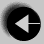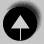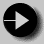## A Weight Conversion Program

```
#include <stdio.h>
#define KILOS_PER_POUND .45359
main()
{       int pounds;

printf(" US lbs      UK st. lbs       INT Kg\n");

for(pounds=10; pounds < 250; pounds+=10)
{       int stones = pounds / 14;
int uklbs = pounds % 14;
float kilos = pounds * KILOS_PER_POUND;
printf("   %d           %d   %d        %f\n",
pounds, stones, uklbs, kilos);
}
}```

Again notice that the only function is called main.

int pounds; Creates a variable of integer type called pounds.

float kilos; Creates a floating point variable (real number) called kilos.

#define KILOS_PER_POUND .45359
defines a constant called KILOS_PER_POUND. This definition will be true throughout the program. It is customary to use capital letters for the names of constants, since they are implemented by the C preprocessor.

for(pounds=10; pounds < 250; pounds+=10)
This is the start of the loop. All statements enclosed in the following curly brackets will be repeated. The loop definition contains three parts separated by semi-colons.

• The first is used to initialise variables when the loop is entered.
• The second is a check, when it proves false, the loop is exited.
• The third is a statement used to modify loop counters on each loop iteration after the first.

The effect of pounds += 10 is to add 10 to the value of the variable pounds. This is a shorthand way of writing pounds = pounds + 10.

The printf statement now contains the symbols %d and %f. These are instructions to print out a decimal (integer) or floating (real) value. The values to be printed are listed after the closing quote of the printf statement. Note also that the printf statement has been split over 2 lines so it can fit onto our page. The computer can recognise this because all C statements end with a semicolon.Go to A very simple programGo to IndexGo to Weight conversion table